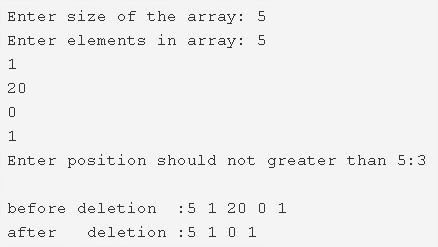# C Program To Delete An Element From An Array At Specified Position | C Programs

C program to delete an element from an array at a specified position – In this article, we will explain the various methods to delete an element from an array at a specified position in C programming.

Suitable examples and sample programs have also been added so that you can understand the whole thing very clearly. The compiler has also been added with which you can execute it yourself.

The methods used in this piece are as follows:

• Using Standard Method
• Using Function

An array is a sequential arrangement of quite a few elements in any order specified in a horizontal fashion.

The locations of the elements of an array are denoted by pointers.As you can see as per the image uploaded above, enter the size of the array.

The size of the array in case of the example is entered as 5.

After that, enter the elements in that array.

The elements here are as follows:

• 5
• 1
• 20
• 0
• 1

Then, you need to enter the position of the element that you want to delete. Make sure that the position number is not larger than the number of elements.

The position ‘3’ is selected for the purpose of deletion.

After the deletion, the array becomes like this:

5 1 0 1

Thus, the multiple means to do the same in C programming are as follows:

## Using Standard Method

1. Read the array size and initialize the value to the variable n.

2) Read the elements using scanf statement and store the elements  into the array as scanf(“%d”,&a[i] ) using for loop for(i=0;i<n;i++).

3) Read the entered position which is not greater than array size using scanf statement and store that value into the variable index.

4) If both the conditions index<=array size and index>0 are true then

a) Print the array elements using print() before deletion of an element from the array.

b) Copy the element of a[i+1] to a[i]. Repeat this step until all iterations of for loop for(i=index-1;i<n;i++). Here i points the index which is the position of deleted element-1. Here, we are moving the elements which are right to the deleted element, to one position left.

c) Print the array elements after deletion of an element from the array using the print() function.

5) If the index  is >n or index<0 then main() prints “invalid input”.

Output:

### Using Function

1. If the entered index is <=n and index>0 then main() calls the newinsertion() function to delete an element from a particular position by passing an array,array size,index as arguments.

2) The function newinsertion() will copy the element of a[i+1]  to a[i] until all iterations of for loop with the structure for(i=index-1;i<n-1;i++).

3) Print the array elements using print(a,n-1) function after deletion of an element from the array. After deletion the size of the array will be decreased by 1.

Output:

x

## C Program To Print Number Of Days In A Month | Java Tutoring

C program to input the month number and print the number of days in that ...